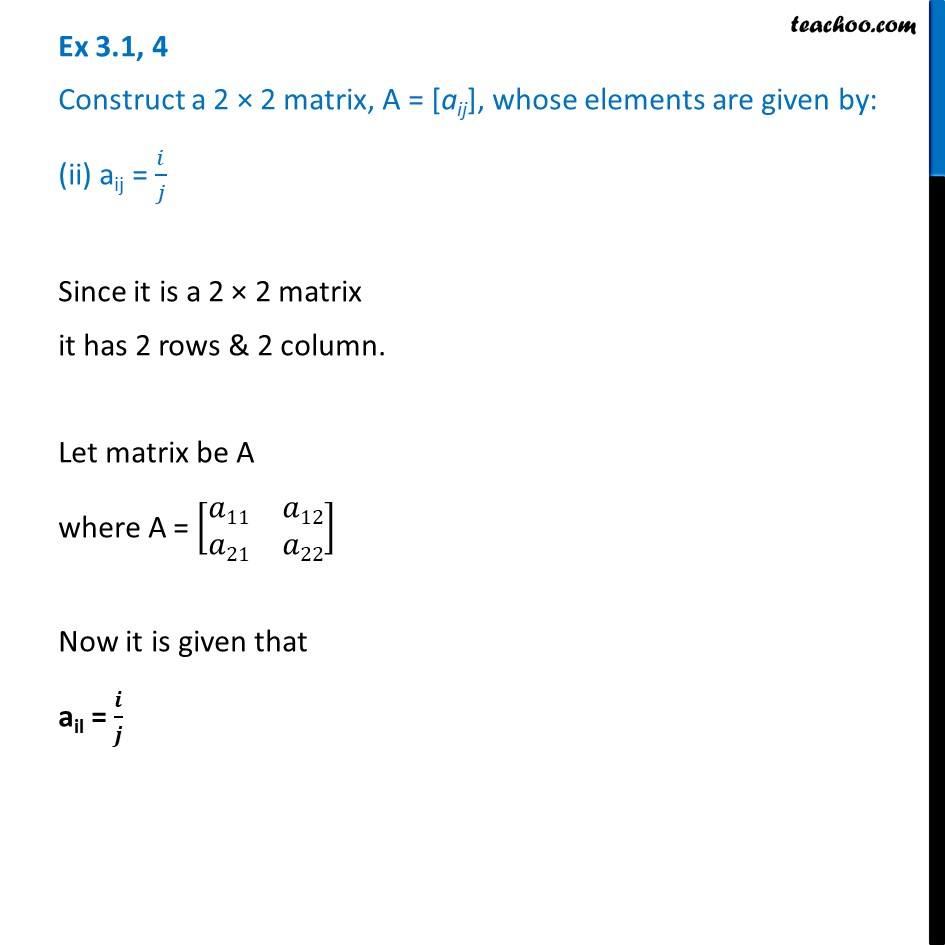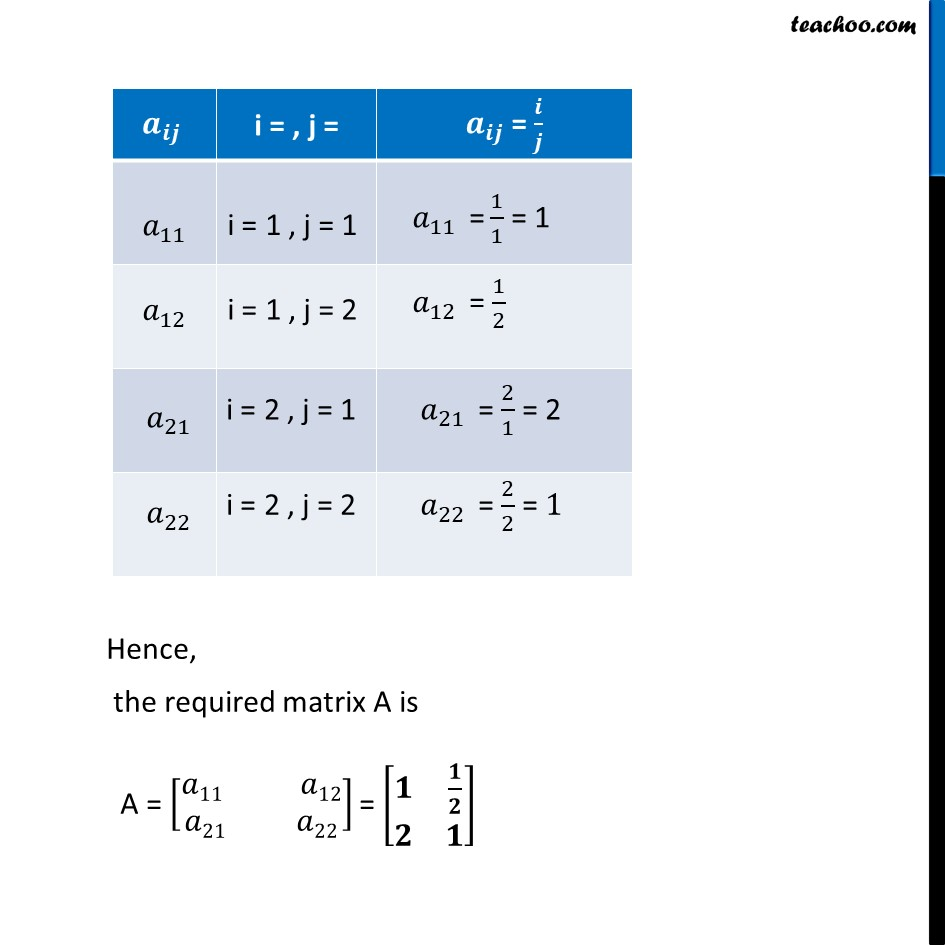Ex 3.1

Chapter 3 Class 12 Matrices
Serial order wiseLearn in your speed, with individual attention - Teachoo Maths 1-on-1 Class

### Transcript

Ex 3.1, 4 Construct a 2 × 2 matrix, A = [aij], whose elements are given by: (ii) aij = 𝑖/𝑗 Since it is a 2 × 2 matrix it has 2 rows & 2 column. Let matrix be A where A = [■8(𝑎11&𝑎12@𝑎21&𝑎22)] Now it is given that ail = 𝒊/𝒋 Hence, the required matrix A is A = [█(𝑎11 𝑎12@𝑎21 𝑎22)] = [■8(𝟏&𝟏/𝟐@𝟐&𝟏)]